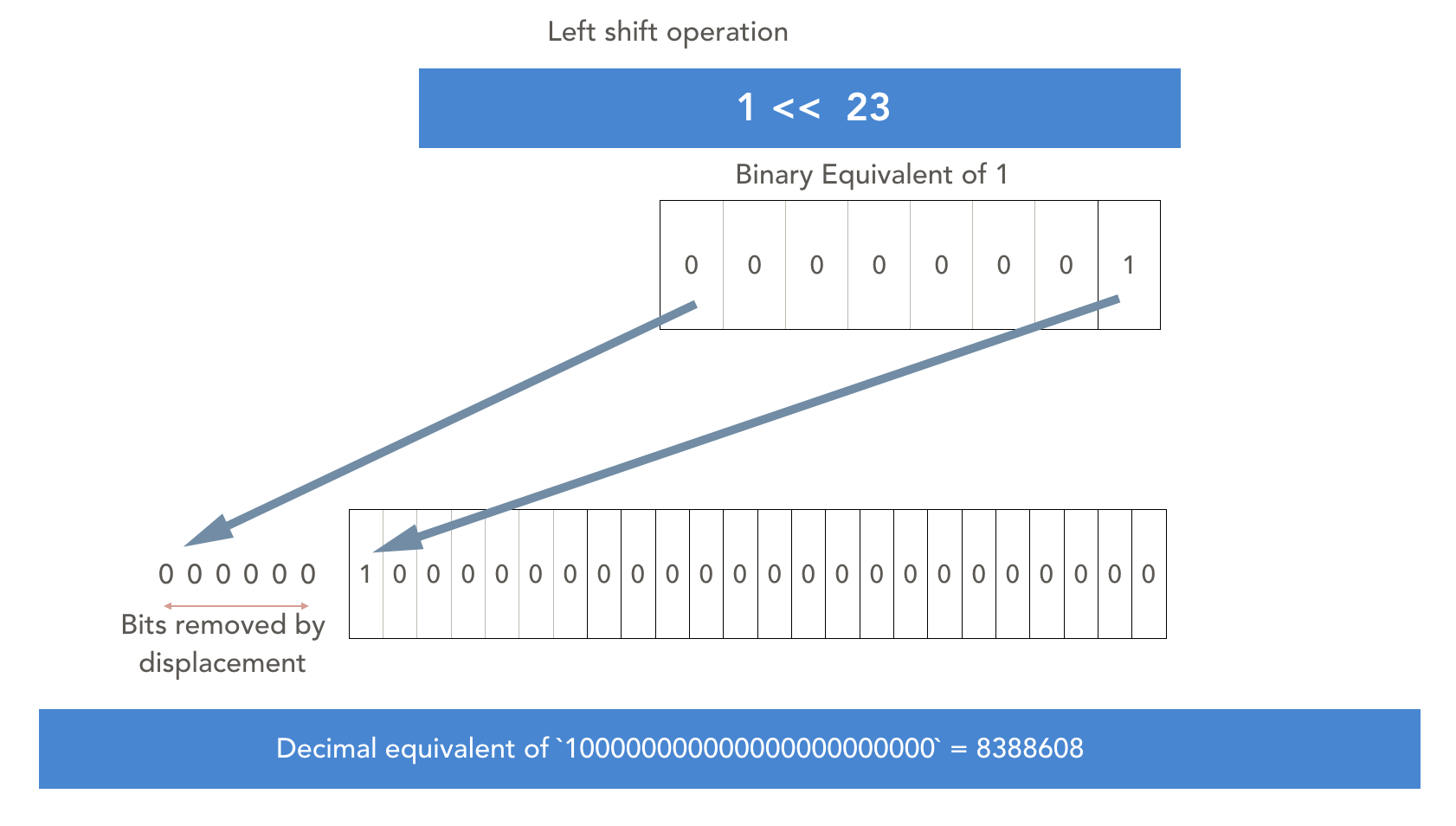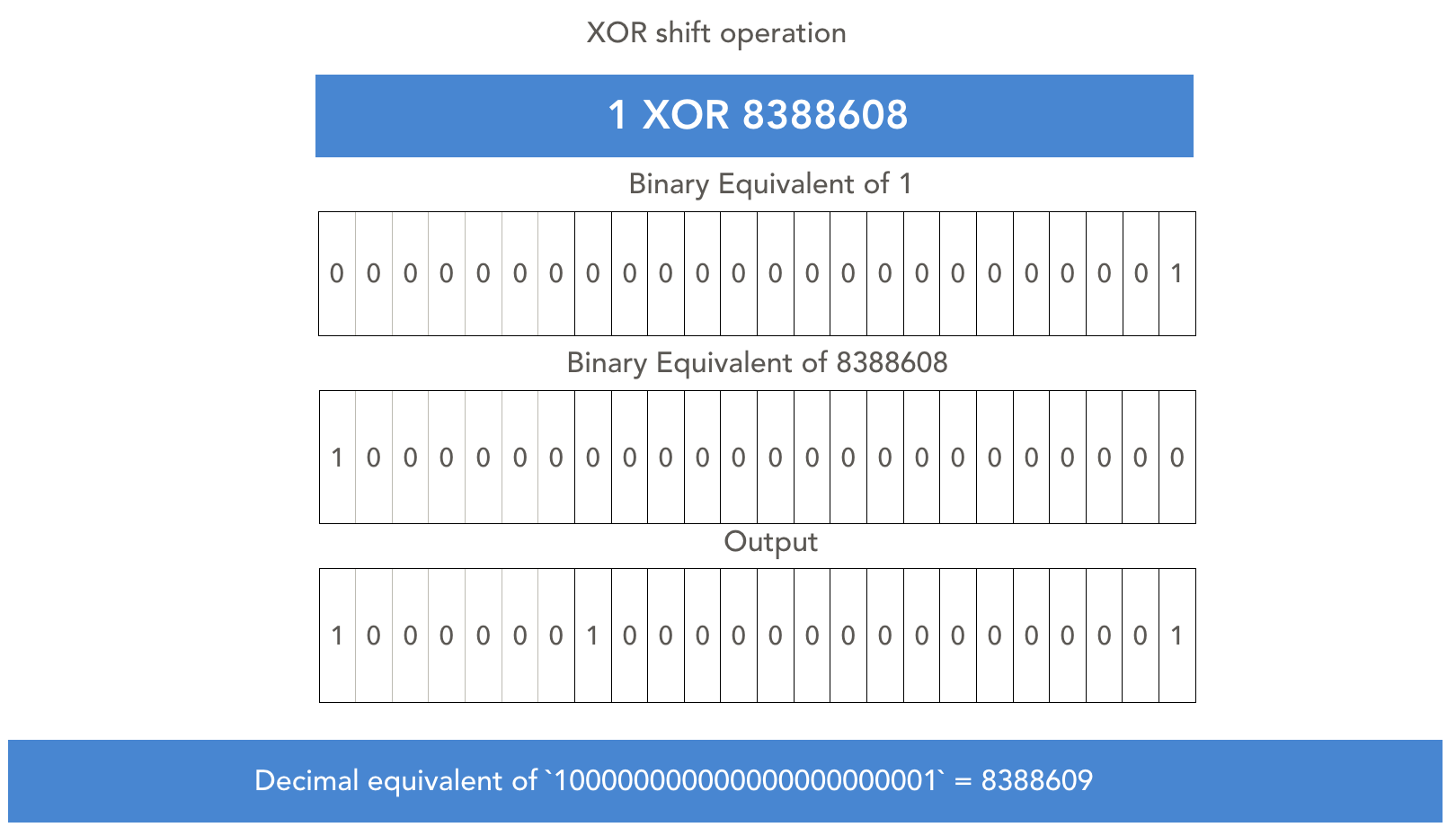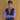# Understanding the math behind Math.random() function

July 13, 2020Photo by fotografierende from Pexels

• Introduction
• If you are a web developer then I am pretty sure you must have used or come across Math.random() in JavaScript at least once. Most of us know that it gives us back a Pseudo-Random Number viz. a number which might seem random but at some level is deterministic based on the function used.
• Research
• So the first article which I came across about this function was from Chrome’s V8 engine’s blog on the implementation of Math.random() which goes into detail about the algorithms used to generate random number in the V8 engine.
• Deep Dive - Now the interesting thing to realise here is Javascript language does not have a specific implementation of this function by itself. There are broad specs specified by ECMAScript, based on which each browser can have thier own implementation for generating random numbers. - Initially, most of the browsers used to have some variation of popular pseudo-random number generation algorithms in their internal engines. In 2015, all the browsers shifted from older algorithms to a standard algorithm called xorshift128+(Adding `+` at the end definitely makes it sound cool 😌).
- If we go through the actual c implementation of the algorithm it looks like as followed:
`````` uint64_t state0 = 1;
uint64_t state1 = 2;
uint64_t xorshift128plus() {
uint64_t s1 = state0;
uint64_t s0 = state1;
state0 = s0;
s1 ^= s1 << 23;
s1 ^= s1 >> 17;
s1 ^= s0;
s1 ^= s0 >> 26;
state1 = s1;
return state0 + state1;
}``````
• Now as a web developer it might seem a bit daunting task to understand this algorithm in C language so let’s port the same implementation to Javascript to understand it better:
``````// Javascript implementation of xorshift128+
let state0 = 1; // Initial seed
let state1 = 2; // Initial seed
function xorshift() {
let s1 = state0;
let s0 = state1;
state0 = s0;
s1 ^= s1 << 23;  // Left shift and XOR operation
s1 ^= s1 >> 17;  // Right shift and XOR operation
s1 ^= s0;
s1 ^= s0 >> 26;  // Right shift and XOR operation
state1 = s1;
return state0 + state1;
}
console.log(xorshift());``````
• The above code written in Javascript looks a bit better but still needs a more explanation. So let’s deep dive into it step by step…
• The first two lines of the algorithm are pretty simple to understand. We initialise two seed values `state0` and `state1`.
• Next from line 4, the real implementation of our algorithm starts. On line 5 and 6 we copy the values of our seed values into variables `s1` and `s0` for temporary manipulations. Next, on line 7 we copy the value of `s0` into `state0` variables. \ At this point if we log the values of all variables they will be:\ `state0=2, state1=2, s0=2, s1= 1`
• Now we come to the most important part of the algorithm…\ `Line 8``:``s1 ^= s1 << 23; // SHIFT`
• Here first, we are performing left shift operation on variable s1, with the syntax `s1 << 23` where the binary equivalent of s1 input value is `1` , is left-shifted by 23 places. Here is a visual representation of how that operation is performed internally:• Next, we perform xor operation on this value with `s1` variable by using `**^=**` syntax. XOR operation compares the binary representations of two numbers and outputs 0 where corresponding bits match and outputs 1 where corresponding bits are different. The XOR operation of s1(of value 1) with the output from previous operation(8388608) is as follows:• In the next 4 lines, similar XOR and shifting operations occur and at line 13 we return the sum values `state1` and `state2`. One important thing to keep in mind is it is the initial seed values which are actually changing, to generate the random values.
• The numbers generated by xorshift128+ aren’t actually random, they’re only relatively evenly distributed over the expected range of values.
• Now how the seed values are selected is dependant on the browsers and underlying engine implementations based on which the range of random values differ.
• Conclusion
• In a nutshell, all the algorithm is really doing here is taking some input, doing some binary operations on them, and giving us a random number. Although the output is quite predictable over long terms but seems pretty random for our day to day use cases.
• References : This concept and implementation were originally covered by: Daniel Simmons on Hackernoon BlogBlog by Saurabh Mhatre
Follow me on Twitter